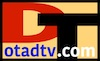Tower Locator Blog# The Decibels (dB) Scale

## dB - decibel

Signal loss or gain is commonly measured in power decibels (dB), and is the power ratio (watts out / watts in) on a base 10 logarithmic (dB) scale. Minus three decibels (-3 dB) has a power ratio of about half (0.5012), plus three decibels (3 dB) is almost double (1.995) power. Signal loss is always a negative (-) dB, signal gain is a positive (+) dB, 0 dB is no loss or gain.

= = dB 10 log10(Pout / Pin) Pout / Pin 10dB/10

 dB ratio Convert dB's and ratio
 Pout = output signal power in watts. Pin = input signal power in watts. Pout = Pin x 10dB/10 Pin = Pout / 10dB/10

## dBm - Signal Power

Signal power is commonly measured in watts or dBm - decibels above or below one milliwatt (1 mW). A negative dBm is dB's below a milliwatt, a positive dBm is dB's above a milliwatt, 0 dBm = 1 mW. The dBm unit is more convenient than watts when dealing with radio, TV, or radar wave signal levels.

 dBm = 10 log10 (mW) mW = 10(dBm/10)

 dBm dBmto watts
 kilowatts (kW) watts (W) milliwatts (mW) microwatts (uW) wattsto dBm

 dBm - decibels above or below 1 mW µW - microwatts = 0.000 001 W mW - milliwatts = 0.001 W W - watts = 1 W kW - kilowatts = 1000 W

Television broadcast signals are typically between 10 kW (70 dBm) to 1000 kW (90 dBm). Receive signals are between -5 dBm (316 µW) to -65 dBm (0.0003 µW).Over-the-Air Digital TV (OTA DTv) The Decibels (dB) Scale © Copyright 2018